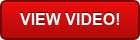Published under: data analysis, Data analytics, logistic regression, Regression, Statgraphics, Life Data

Fitting Regression Models Containing Categorical Factors

Join us on December 14th at 11:00AM EST for the next Statgraphics webinar!

This webinar will discuss the use of regression analysis models that include both quantitative and categorical predictive factors. It begins with a discussion of the use of indicator variables in models with a single X and then moves on to multiple regression models. The inclusion of categorical factors in nonlinear regression, logistic regression, and life data regression is also considered.

This webinar will be of interest to anyone who deals with data that is divided into groups and wishes to test whether regression relationships differ significantly between the groups. It will also be of interest to analysts who are developing predictive models that involve both quantitative and non-quantitative factors.